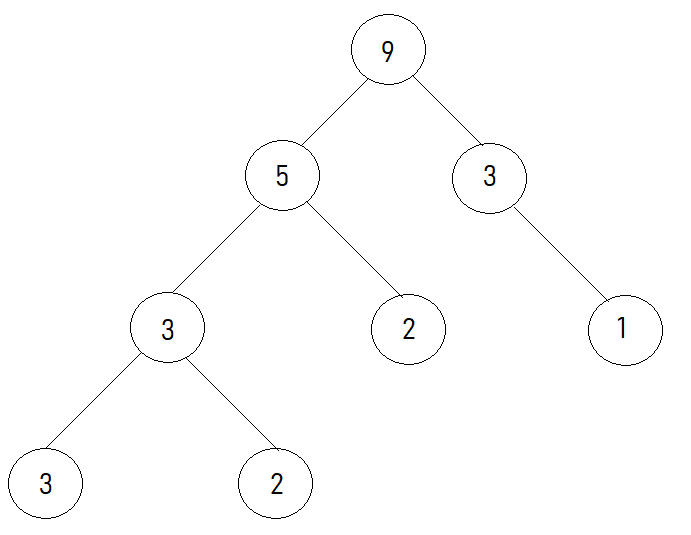17

# Sum root to leaf

Difficulty: EASYContributed By
Ekansh Saxena
Avg. time to solve
10 min
Success Rate
90%

Problem Statement
Suggest Edit

#### For example:

``````       1
/  \
2    3

The root to leaf path 1->2 represents the number 12.
The root to leaf path 1->3 represents the number 13.
``````

#### In the above example,

``````The total sum of all the possible root to leaf paths is 12+13 = 25
``````
##### Note:
``````The output may be very large, return the answer after taking modulus with (10^9+7).
``````
##### Input format
``````The first line of input contains an integer ‘T’ denoting the number of test cases.
The next ‘T’ lines represent the ‘T’ test cases.

The only line of each test case contains the elements of the tree in the level order form separated by a single space.

If any node does not have a left or right child, take -1 in its place. Refer to the example below.
``````

Example:

``````Elements are in the level order form. The input consists of values of nodes separated by a single space in a single line. In case a node is null, we take -1 in its place.

For example, the input for the tree depicted in the below image would be :
```````````` 1
2 3
4 -1 5 6
-1 7 -1 -1 -1 -1
-1 -1

Explanation :
Level 1 :
The root node of the tree is 1

Level 2 :
Left child of 1 = 2
Right child of 1 = 3

Level 3 :
Left child of 2 = 4
Right child of 2 = null (-1)
Left child of 3 = 5
Right child of 3 = 6

Level 4 :
Left child of 4 = null (-1)
Right child of 4 = 7
Left child of 5 = null (-1)
Right child of 5 = null (-1)
Left child of 6 = null (-1)
Right child of 6 = null (-1)

Level 5 :
Left child of 7 = null (-1)
Right child of 7 = null (-1)

The first not-null node (of the previous level) is treated as the parent of the first two nodes of the current level. The second not-null node (of the previous level) is treated as the parent node for the next two nodes of the current level and so on.

The input ends when all nodes at the last level are null (-1).
``````
##### Note :
``````The above format was just to provide clarity on how the input is formed for a given tree.

The sequence will be put together in a single line separated by a single space. Hence, for the above-depicted tree, the input will be given as:

1 2 3 4 -1 5 6 -1 7 -1 -1 -1 -1 -1 -1
``````
##### Output format:
``````For each test case, return the total sum of all the possible root to leaf paths.

Output for each test case must be in a separate line.
``````
##### Note :
``````You do not need to print anything, it has already been taken care of. Just implement the given function.
``````
##### Constraints:
``````1 <= T <= 50
1 <= N <= 3000
1 <= nodeValue <= 9

Where ‘N’ is the number of nodes in the tree and nodeValue denotes data contained in the node of a binary tree.

Time limit: 1 sec
``````
##### Sample input 1:
``````2
9 5 3 3 2 -1 1 3 2 -1 -1 -1 -1 -1 -1 -1 -1
1 3 2 2 1 4 5 -1 -1 1 -1 1 2 -1 6 -1 -1 -1 -1 -1 -1 -1 -1
``````
##### Sample output 1:
``````20948
5182
``````
##### Explanation of sample input 1:
``````Test case 1:
The above tree looks like:-
````````````All possible paths from root to leaf are:
9->5->3->3 giving number 9533
9->5->3->2 giving number 9532
9->5->2 giving number 952
9->3->1 giving number 931

The total sum of all possible root to leaf paths numbers is 9533+9532+952+931 = 20948 and 20948 mod 10^9 + 7 = 20948

Test case 2:
All possible paths from root to leaf are:
1->3->2 giving number 132
1->3->1->1 giving number 1311
1->2->4->1 giving number 1241
1->2->4->2 giving number 1242
1->2->5->6 giving number 1256
The total sum of all possible root to leaf paths numbers is 132+1311+1241+1242+1256 = 5182
``````
##### Sample input 2:
``````2
1 3 4 1 2 -1 6 -1 -1 -1 -1 -1 -1
1 2 2 5 3 -1 1 -1 -1 3 -1 -1 -1 -1 -1
``````
##### Sample output 2:
``````409
1479
``````Console